RXTE Helpdesk/FAQ RXTE What's New HEASARC Site Map

# The ABC of XTE A Time Tutorial

## Postscript version of this chapter

NOTE: The time stamps present in RXTE FITS tables as generated by XFF are in TT.

## Introduction

This chapter aims at collecting brief definitions of the various time systems in use, as well as simple formulae for doing transformations. Information was gleaned from a number of sources, like the Explanatory Supplement to the Astronomical Ephemeris (1992), a paper by Soffel and Brumberg in Celestial Mechanics and Dynamical Astronomy 52, 355 (1991), and several papers in the Proceedings of the 6th European Frequency and Time Forum (ESA SP-340, 1992).

## Time and Date Systems

1. TAI (International Atomic Time): Based on the SI second and derived from a large number of clocks all over the world. Analyzed and published by by BIPM. Reduced to mean sea level.

2. UTC (Universal Time, Coordinated): Based on the SI second, it knows days of 86400 and 86401 seconds. The latter are days with a so-called leap second. The objective is to keep UTC within a second of UT1, which is based on the earth's rotation. The offset from TAI is always an integer number of seconds (the accumulated leap seconds).

3. TT (Terrestrial Time): An idealized time, for all practical purposes based on TAI, but with a constant offset to provide continuity with Ephemeris Time. Defined on the rotating geoid.

4. TB (Barycentric Time): Also known as TDB (Barycentric Dynamical Time). This is basically TT transformed to the solar system barycenter.

5. TCG (Geocentric Coordinate Time): A properly relativistic coordinate time, referred to the geocenter. The coordinate time equivalent of TT.

6. TCB (Barycentric Coordinate Time): The coordinate time equivalent of TB.

7. JD (Julian Date): Number of days since Greenwich mean noon on January 1, 4713 B.C. Note that JD may be used in conjunction with all of the above time systems; whenever the required accuracy dictates this, the time system used should be indicated: e.g., JD (TT).

8. MJD (Modified Julian Date): Equals JD - 2400000.5. The same note applies as for JD concerning the time system in use. We shall continue to use MJD notwithstanding the IAU's recent decision not to recognize MJD anymore as an official time unit.

## Useful Relations

1. JD - MJD: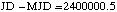2. TT - TAI: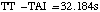3. TAI - UTC: Since 1972, an integer number of seconds. Please consult the IERS UTC document for the history of the leap seconds.

4. TCB - TCG: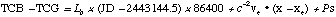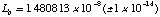The vectors xe and ve denote the barycentric position and velocity of the earth's center of mass, and x is the barycentric position of the observer. The quantity P represents periodic terms which can be evaluated using analytical formulae. For observers on the Earth's surface, these are diurnal, with a maximum amplitude of 2.1 microseconds; for spacecraft, they presumably depend solely on the orbit.

The origins of coordinate times have been arbitrarily set so that these times all coincide with TT at the geocenter of 1977.0 (TAI).

5. TB - TT: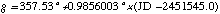6. TCG - TT: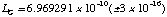7. TCB - TB: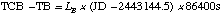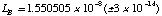## Other Useful Formulae

1. Relation between time on the geoid (t') and time on a Low Earth Orbit spacecraft (tL):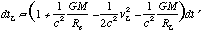This approximates to:At an altitude of 500 km, the clock would loose approximately 8.5 ms per year, unless its frequency were adjusted.

## RXTE FITS Tables

The time stamps presented by RXTE FITS tables as generated by XFF are in TT.

It has been agreed that the Spacecraft Clock Seconds (SCCS), or Mission Elapsed Time (MET), will represent true elapsed seconds since January 1, 1994, at 0h0m0s UTC, which corresponds to MJD = 49353.0 (UTC).

Raw time stamps in the FITS tables created by XFF will be on this (SCCS or MET) time system. However, FITS times are to be taken as the sum of a Time value and the values of the MJDREF and TIMEZERO keywords. The MJDREF keyword will incorporate the transformation from UTC, via TAI, to TT (equaling an offset of 60.184 s). Thus, for January 1, 1994, at 0h UTC:

MJD (Jan 1, 1994, 0h UTC) = 49353.0 (UTC) = 49353.000696574074 (TT)

The TIMEZERO keyword will provide a nominal clock correction that ensures an absolute accuracy of 60 µs for the time stamps. The goal is to provide reasonably accurate absolute time with a minimum effort. Generically, the value of TIMEZERO may be described as "the fractional part of UTCF".

[UTCF is an institutional dinosaur that can be found in the telemetry. Its purpose is to provide a conversion to UTC: when the sum of SCCS and UTCF is divided by 86400, the integer part of the result provides the number of UTC days since January 1, 1993, at 0h UTC, while the fractional part indicates the current UTC. Thus, UTCF is the sum of the number of seconds in 1993 (31,536,001) and the clock correction, minus the number of leap seconds since January 1, 1993.]

To summarize the times in the FITS tables:

• Raw time stamps are in SCCS = MET.

• Time stamps plus TIMEZERO provide time in TAI, as seconds since January 1, 1994, at 0h 0m 28s (TAI).

• Time stamps plus TIMEZERO plus MJDREF provides absolute time in TT.

• All RXTE science time stamps refer to the start of the bin, i.e. TIMEPIXR=0.0. In the case of event lists, the EDS rounds time values downward to the nearest time bin edge. In other words, the least significant time bits of event times are truncated by the EDS.

• All time-related quantities are in seconds, except for MJDREF which is in days.

Thus, to compute the modified Julian days (MJD) from raw XTE FITS files, referred to the TT time system, one should use the following formula,
MJD(TT) = (MJDREFI+MJDREFF)+(TIME+TIMEZERO+FINECLOCK)/86400
As noted above, this will compute the time at the start of light curve bin or the truncated event time. To compute a time which is properly centered on each time bin, use this modified expression:
MJD(TT) = (MJDREFI+MJDREFF)+(TIME+TIMEZERO+FINECLOCK+(0.5-TIMEPIXR)*TIMEDEL)/86400
where TIMEPIXR is always 0 for RXTE data, and TIMEDEL is the time resolution of the event data, or bin size for light curve data.

In order to recover the most precise absolute times, the fine clock corrections found in the tdc.dat file must be applied, which is the FINECLOCK term listed in the formulae above. The fine clock corrections are based on ground station estimates of the RXTE clock offset and drift rate. These values are not a value which is immediately available in the observation data, but rather are estimated after the fact by the RXTE GOF and delivered approximately monthly. See the RXTE Absolute Timing page for more details about fine clock corrections, and which tools incorporate these. See the Timing Digest for a figure of fine clock corrections as a function of time. The maximum attainable absolute time precision, using the tdc.dat file corrections, is about 2.5 microseconds for most of the mission (ref. Jahoda et al. 2006, ApJS, 163, 401).

It may be possible to reach even higher accuracies, to the level of a few microseconds, but this will require a knowledgeable guest observer fine-tuning the clock corrections "by hand". No SOC support will be extended for this, beyond providing the guest observer with all data readily available to the SOC.

The relevant FITS table keywords are summarized below:

```  Keyword      Value              Unit   Comment

TTYPE1      'TIME'                s    Column 1 contains raw time in SCCS
TIMESYS     'TT'                       Defines TT as fundamental time system
MJDREF      49353.000696574074    d    1994.0 (UTC) in TT
TIMEZERO    frac(UTCF)            s    Clock correction
```
Various transformations between MET, TT, and UTC in a variety of formats (seconds, calendar date/time, year-day-time, JD, MJD) can be performed using the xTime web tool.

## Barycentering

For high precision timing work, one often has to account for the fact that the spacecraft and earth are moving through the solar system. Since the speed of light is finite, the arrival time of a photon from a target will be delayed or advanced, depending on the position of the observatory within the solar system. The amount of delay or advance varies appxroximately sinusoidally with time, with a period of one year and an amplitude of about 8 minutes. Any astrophysical observed variations from the target will be advanced or delayed by the same amount.

Removing these observatory-related variations is known as barycentering. Photon arrival times are adjusted so they represent the effective arrival time to a ficticious observer, located at the solar system barycenter. The solar system time scale is known as Barycentric Time. The software tasks 'fxbary', 'faxbary' and 'barycorr' are capable of performing this correction on RXTE data. The adjusted barycentered times will be written to either the 'TIME' or 'BARYTIME' column, depending on the options used.

After barycentering, the 'TIME' or 'BARYTIME' column contains the photon arrival time in TDB seconds, since the reference epoch, MJDREF, now also expressed in the TDB system. Thus, to compute the MJD time from barycentered files, referred to the TDB system, one should use one of the following formulae,
MJD(TDB) = (MJDREFI+MJDREFF)+(BARYTIME+TIMEZERO)/86400    (or)
MJD(TDB) = (MJDREFI+MJDREFF)+(TIME+TIMEZERO)/86400
depending on task the settings used. If the TIMEZERO keyword is not present, the default is zero.

After barycentering, the contents of the FITS table will be transformed, as follows:

```  Keyword      Value              Unit   Comment

TTYPE1      'TIME' or 'BARYTIME'  s    Column name depends on task settings
TIMESYS     'TDB'                      Time system now in Barycentric Time
MJDREF      49353.000696574074    d    1994.0 (UTC) in TDB
TIMEZERO    0 or undefined        s    Should have been absorbed into time column
```

## Changes

• Original - written by Arnold Rots
• 2001-02-21 - discussion of fine clock corrections - Padi Boyd
• 2009-01-17 - discussion of barycentering, 3 usec absolute timing - C. B. Markwardt
• 2010-05-12 - add discussion of TIMEPIXR and compute time at center of time bin - C. B. Markwardt